# 美景本天成，妙笔偶得之——“妙笔”是怎样炼成的？

2022/02/11 04:02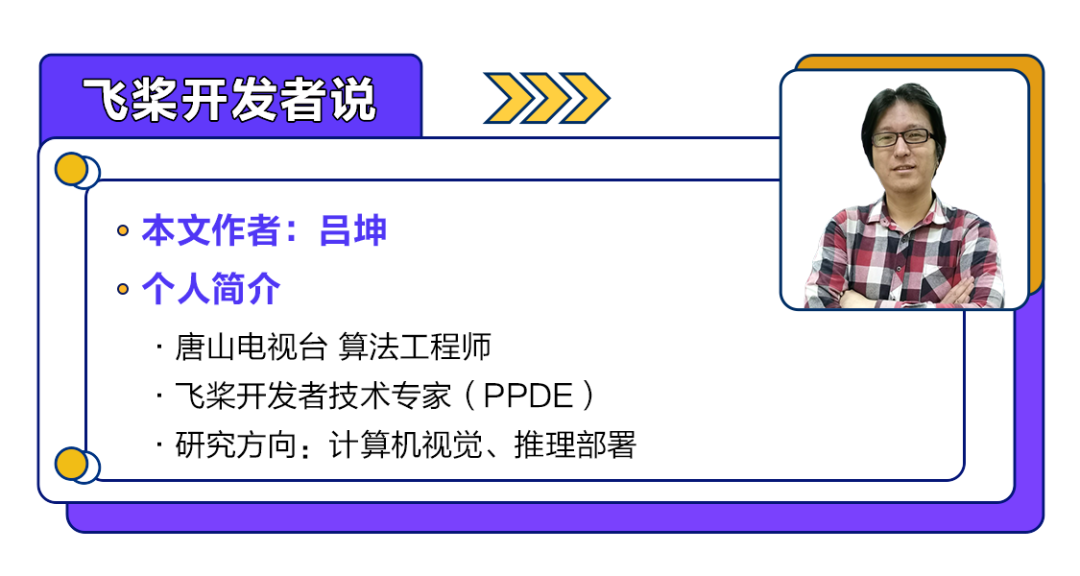1.多尺度判别器

（Multi-scale discriminators）

# Multi-scale discriminators 判别器代码
class MultiscaleDiscriminator(nn.Layer):
def __init__(self, opt):
super(MultiscaleDiscriminator, self).__init__()

for i in range(opt.num_D):
sequence = []
feat_size = opt.crop_size
for j in range(i):
sequence += [nn.AvgPool2D(3, 2, 1)]
feat_size = np.floor((feat_size + 1 * 2 - (3 - 2)) / 2).astype('int64') # 计算各个判别器输入的缩放比例
opt_downsampled = copy.deepcopy(opt)
opt_downsampled.crop_size = feat_size
sequence += [NLayersDiscriminator(opt_downsampled)]
sequence = nn.Sequential(*sequence)

def forward(self, input):
output = []
for layer in self._sub_layers.values():
output.append(layer(input))
return output

2.逐渐精细化的生成器

（Coarse-to-fine generator）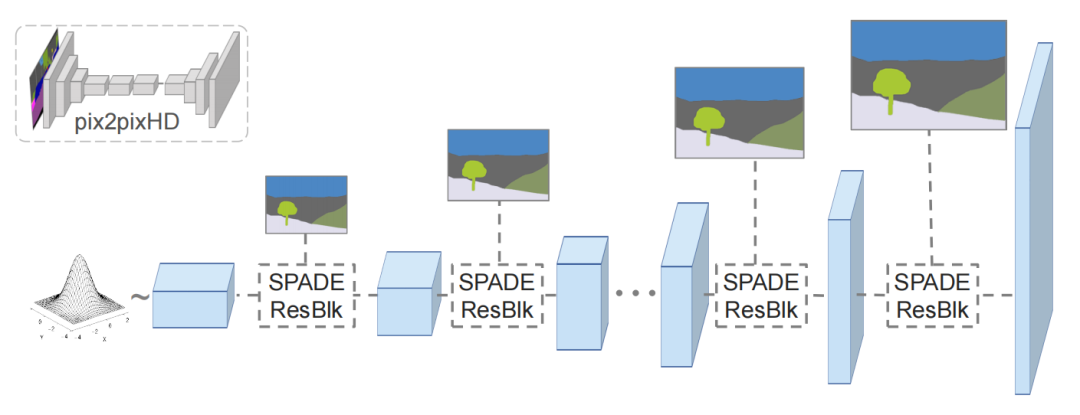# Coarse-to-fine generator 生成器代码
def __init__(self, opt):

self.opt = opt
nf = opt.ngf
self.sw, self.sh = self.compute_latent_vector_size(opt)

if self.opt.use_vae:
self.fc = nn.Linear(opt.z_dim, 16 * opt.nef * self.sw * self.sh)
else:
self.fc = nn.Conv2D(self.opt.semantic_nc, 16 * nf, 3, 1, 1)

self.G_middle_0 = SPADEResnetBlock(16 * nf, 16 * nf, opt)
self.G_middle_1 = SPADEResnetBlock(16 * nf, 16 * nf, opt)

self.up_0 = SPADEResnetBlock(16 * nf, 8 * nf, opt)
self.up_1 = SPADEResnetBlock(8 * nf, 4 * nf, opt)
self.up_2 = SPADEResnetBlock(4 * nf, 2 * nf, opt)
self.up_3 = SPADEResnetBlock(2 * nf, 1 * nf, opt)

final_nc = nf

if opt.num_upsampling_layers == 'most':
self.up_4 = SPADEResnetBlock(1 * nf, nf // 2, opt)
final_nc = nf // 2

self.conv_img = nn.Conv2D(final_nc, 3, 3, 1, 1)

self.up = nn.Upsample(scale_factor=2)

def forward(self, input, z=None):
seg = input
if self.opt.use_vae:
x = self.fc(z)
x = paddle.reshape(x, [-1, 16 * self.opt.nef, self.sh, self.sw])
else:
x = F.interpolate(seg, (self.sh, self.sw))
x = self.fc(x)

x = self.up(x)
x = self.G_middle_0(x, seg)

if self.opt.num_upsampling_layers == 'more' or \
self.opt.num_upsampling_layers == 'most':
x = self.up(x)

x = self.G_middle_1(x, seg)

x = self.up(x)
x = self.up_0(x, seg)
x = self.up(x)
x = self.up_1(x, seg)
x = self.up(x)
x = self.up_2(x, seg)
x = self.up(x)
x = self.up_3(x, seg)

if self.opt.num_upsampling_layers == 'most':
x = self.up(x)
x = self.up_4(x, seg)

x = self.conv_img(F.gelu(x))
x = F.tanh(x)

return x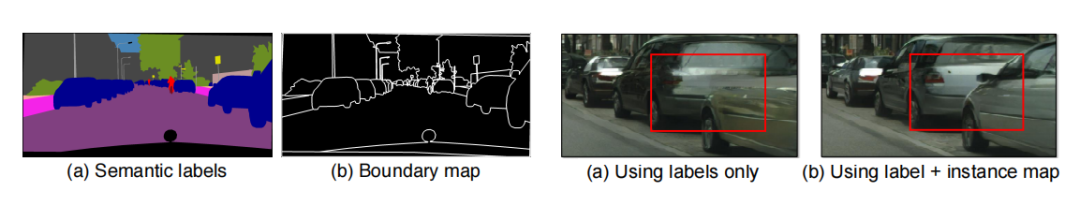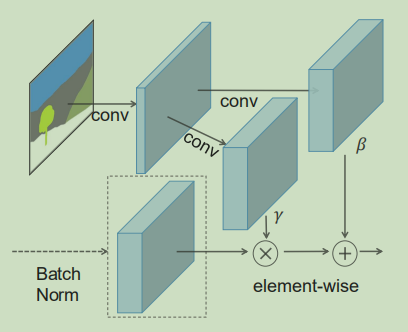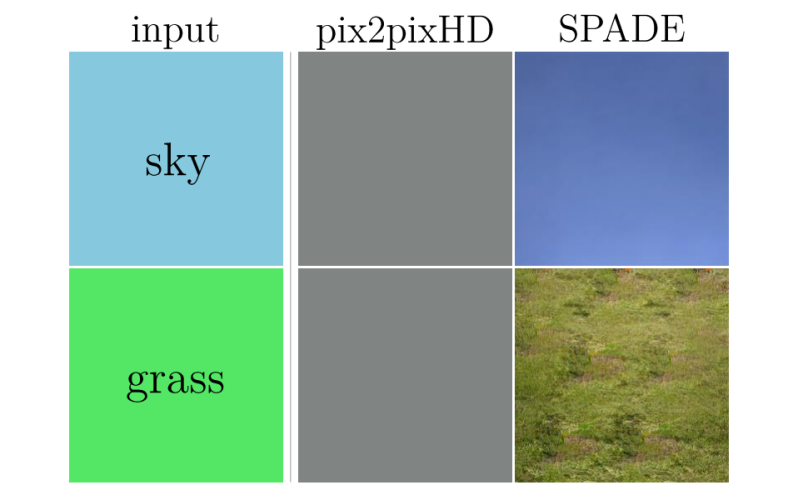# SPADE空间自适应归一化模块代码
def __init__(self, config_text, norm_nc, label_nc):

param_free_norm_type = str(parsed.group(1))
ks = int(parsed.group(2))

self.param_free_norm = build_norm_layer(param_free_norm_type)(norm_nc) # 此处理须关闭归一化层的自适应参数

# The dimension of the intermediate embedding space. Yes, hardcoded.
nhidden = 128

pw = ks // 2
self.mlp_shared = nn.Sequential(*[
nn.Conv2D(label_nc, nhidden, ks, 1, pw),
nn.GELU(),
])
self.mlp_gamma = nn.Conv2D(nhidden, norm_nc, ks, 1, pw)
self.mlp_beta = nn.Conv2D(nhidden, norm_nc, ks, 1, pw)

def forward(self, x, segmap):
# Part 1. generate parameter-free normalized activations
normalized = self.param_free_norm(x)

# Part 2. produce scaling and bias conditioned on semantic map
segmap = F.interpolate(segmap, x.shape[2:])
actv = self.mlp_shared(segmap)
gamma = self.mlp_gamma(actv)
beta = self.mlp_beta(actv)

# apply scale and bias
out = normalized * (1 + gamma) + beta

return out

SPADE模块首先关掉了归一化层的自适应缩放系数和偏置，然后将缩放后的特征图（以适应前一层不同尺寸的输出）embedding 到 mlp_gamma 卷积层中，然后在分别映射到 gamma 卷积层（缩放）和 beta 卷积层（偏置），这样就完成了“用2d卷积层替换替换标量缩放系数和偏置”的操作，以达到保存“通过BN层的空间信息”的目的。

4.GauGAN的Loss 计算

（hinge Loss、Feat Loss、Perceptual Loss）

GauGAN的多尺度判别器不但集成了多个缩放尺寸的判别器，而且在计算判别 Loss时，不但计算最后一层输出的结果，判别器中间层输出的特征图也参与Loss计算，公式如下：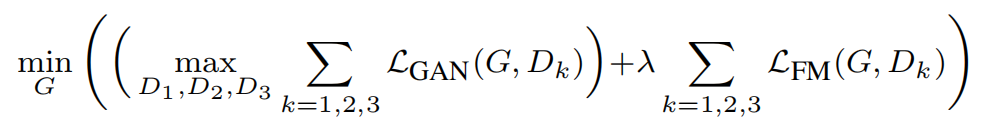Pix2PixHD还使用了ImageNet数据集上预训练的VGG19模型作为额外特征提取器计算Perceptual Loss，用于比对真假图片。与使用判别器中间层输出的特征图计算Loss时不同，使用VGG19中间层特征图计算Loss时要逐层加权，使得模型对高层的语义特征更敏感。

GauGAN的Loss由“对抗损失”、“判别器辅助损失”和“生成器辅助损失” 三部分组成。

①对抗损失采用Hinge Loss

# 判别器对抗损失
df_ganloss = 0.
for i in range(len(pred)):
pred_i = pred[i][-1][:batch_size]
df_ganloss += new_loss
df_ganloss /= len(pred)

dr_ganloss = 0.
for i in range(len(pred)):
pred_i = pred[i][-1][batch_size:]
dr_ganloss += new_loss
dr_ganloss /= len(pred)

# 生成器对抗损失
g_ganloss = 0.
for i in range(len(pred)):
pred_i = pred[i][-1][:batch_size]
new_loss = -pred_i.mean() # hinge loss
g_ganloss += new_loss
g_ganloss /= len(pred)

df_ganloss和dr_ganloss分别是判别假图片和真图片的Loss，g_ganloss是生成器损失。使用hinge loss计算判别器损失时，每次只用部分样本的损失更新梯度，稳定了生成器的更新。因此，后来的改进模型甚至去掉了用于稳定判别器更新的谱归一化层。

②判别器辅助损失使用判别器中间层输出的特征图计算 L1 Loss 加和而成

g_featloss = 0.
for i in range(len(pred)):
for j in range(len(pred[i]) - 1): # 除去最后一层的中间层featuremap
unweighted_loss = (pred[i][j][:batch_size] - pred[i][j][batch_size:]).abs().mean() # L1 loss
g_featloss += unweighted_loss * opt.lambda_feat / len(pred)

③生成器辅助损失使用 VGG19 预训练模型中间层输出的特征图逐层加权计算 L1 Loss 加和而成

g_vggloss = paddle.to_tensor(0.)
if not opt.no_vgg_loss:
rates = [1.0 / 32, 1.0 / 16, 1.0 / 8, 1.0 / 4, 1.0]
_, fake_features = vgg19(resize(fake_img, opt, 224))
_, real_features = vgg19(resize(image, opt, 224))
for i in range(len(fake_features)):
g_vggloss += rates[i] * l1loss(fake_features[i], real_features[i])
g_vggloss *= opt.lambda_vgg

④GauGAN总的损失函数

d_loss = df_ganloss + dr_ganloss

if opt.use_vae:
g_loss = g_ganloss + g_featloss + g_vggloss + g_vaeloss
g_loss.backward(retain_graph=True)
opt_e.step()
else:
g_loss = g_ganloss + g_featloss + g_vggloss
g_loss.backward()
opt_g.step()

1.项目实现中遇到的一些问题

①数据处理

CycleGAN提出的时候曾经吐槽过Pix2Pix这种像素风格迁移模型严重依赖成对的数据集。但是，幸好“图像分割”作为CV深度学习三剑客（图像分类、目标检测、图像分割）之一，有大量的训练数据集和预训练模型可以用到“风格迁移/语义图像合成”任务中。

2.模型改进及正在进行的后续处理

①添加注意力

#### 现在，注意力很流行，但语义图像合成离着上Transfomer还有点遥远，那么就先用21年“上新”的SimAM（Simple, Parameter-Free Attention Module 简单无参注意力模块）试试吧。这个注意力机制借鉴了神经科学理论，使用能量函数评估神经元的重要性。代码如下：

def simam(x, e_lambda=1e-4):
b, c, h, w = x.shape
n = w * h - 1
x_minus_mu_square = (x - x.mean(axis=[2, 3], keepdim=True)) ** 2
y = x_minus_mu_square / (4 * (x_minus_mu_square.sum(axis=[2, 3], keepdim=True) / n + e_lambda)) + 0.5
return x * nn.functional.sigmoid(y)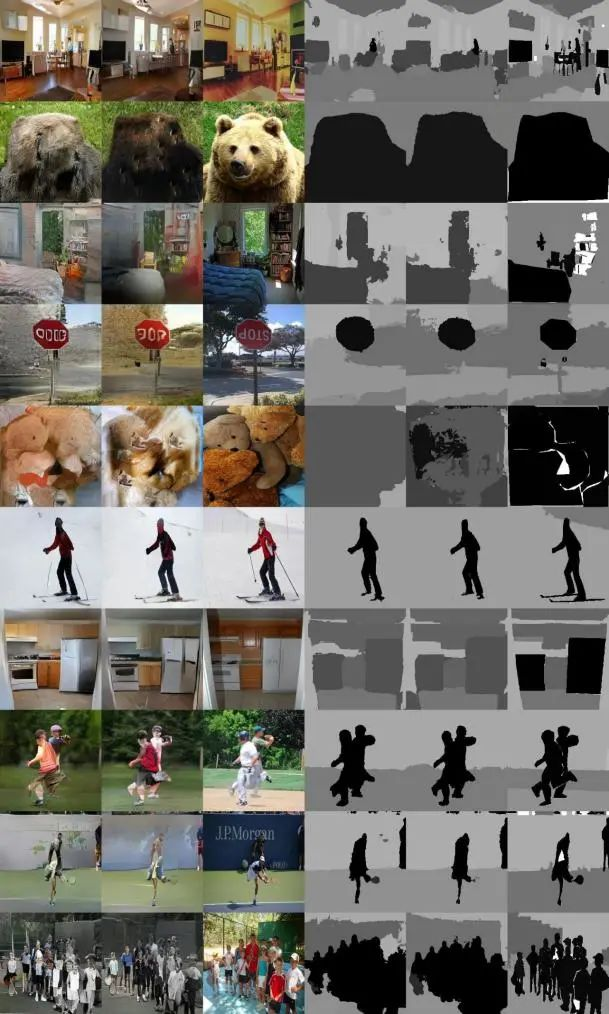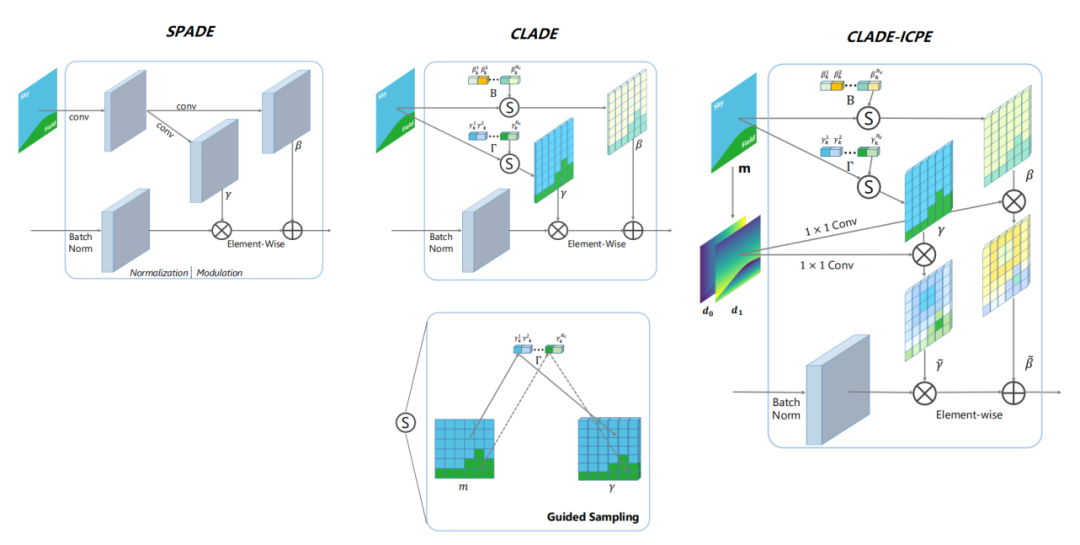#### ③压缩模型

GAN CAT（Compression And Teaching 压缩和蒸馏）

GAN CAT方法的最大特点是：教师生成器TeacherG除了用于蒸馏，还作为模型搜索空间使用，无需训练额外的Supernet模型。模型结构的搜索空间通过InsResBlocks模块实现。裁剪过程同时选择模型结构与通道。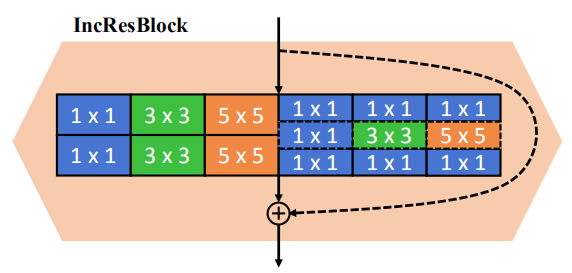CAT方法裁剪使用的阈值（归一化层的缩放因子）根据压缩目标自动求出，无需迭代裁剪过程。蒸馏使用 KA（Kernel Alignment）衡量不同通道数的卷积结构之间的相似性。

OMGD（Online Multi-Granularity Distillation 多粒度在线蒸馏）

OMGD的思路非常清晰，就是用一个更深的模型和一个更宽的模型来进行蒸馏，一图以蔽之：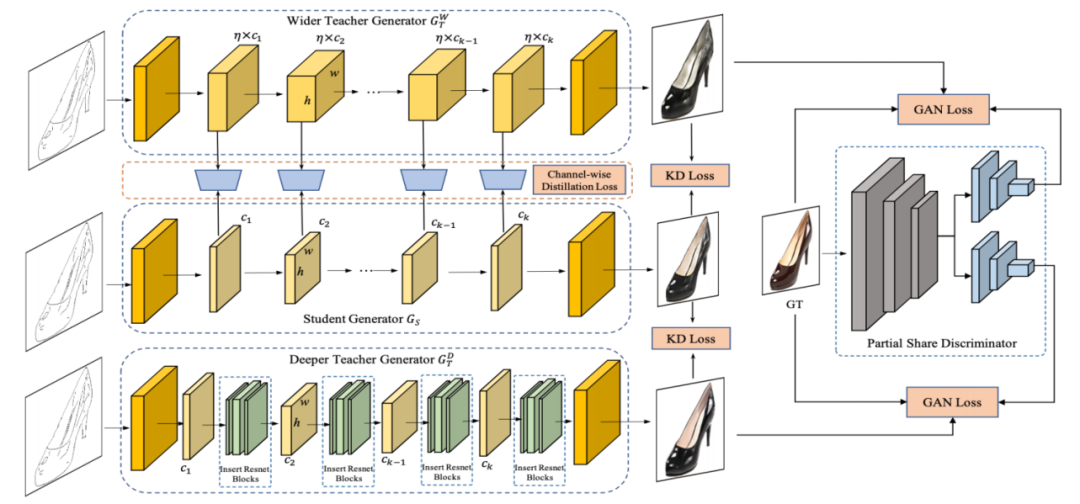OMGD一边训练两个更深、更宽的教师生成器，一边用其进行蒸馏，能够使过程更加稳定，这就是在线。使用深度相同宽度更宽的教师模型进行蒸馏时，loss函数不但比对输出结果，而且对中间层的特征图也使用Structural Similarity (SSIM) Loss进行比对，是以称之为多粒度。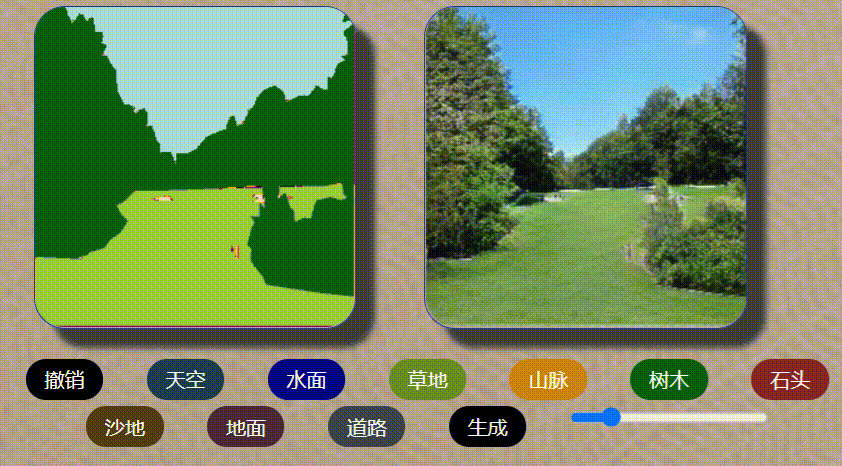①GAN的“风格迁移五部曲”

• 《一文搞懂生成对抗网络之经典GAN》 https://aistudio.baidu.com/aistudio/projectdetail/551962

• 《一文搞懂GAN的风格迁移之Conditional GAN》 https://aistudio.baidu.com/aistudio/projectdetail/644398

• 《一文搞懂GAN的风格迁移之Pix2Pix》 https://aistudio.baidu.com/aistudio/projectdetail/1119048

• 《一文搞懂GAN的风格迁移之CycleGAN》 https://aistudio.baidu.com/aistudio/projectdetail/1153303

https://aistudio.baidu.com/aistudio/projectdetail/1964617

• 《妙笔生画》

https://aistudio.baidu.com/aistudio/projectdetail/2274565

②GAN“前传”

 Park T, Liu M Y, Wang T C, et al. Semantic Image Synthesis With Spatially-Adaptive Normalization[C]// 2019 IEEE/CVF Conference on Computer Vision and Pattern Recognition (CVPR). IEEE, 2019.### 作者的其它热门文章

0
0 收藏

0 评论
0 收藏
0